# Java operators

Operator in java is a symbol that is used to perform some operation.

Example: `+` , `-` , `*`, `/` etc.

##### Java operators has been classified as following categories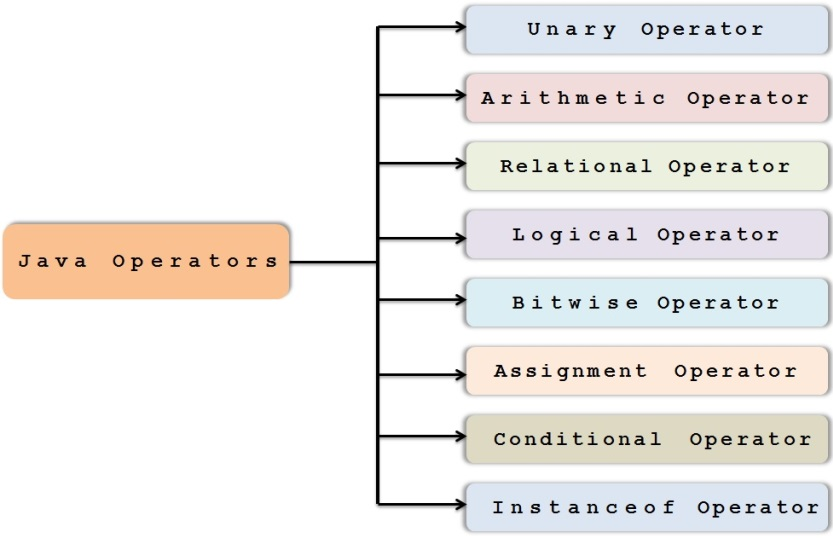##### Unary Operator

The unary operators works on only one operand, they perform various operations such as `incrementing/decrementing` a value by one, negating an expression, or inverting the value of a boolean.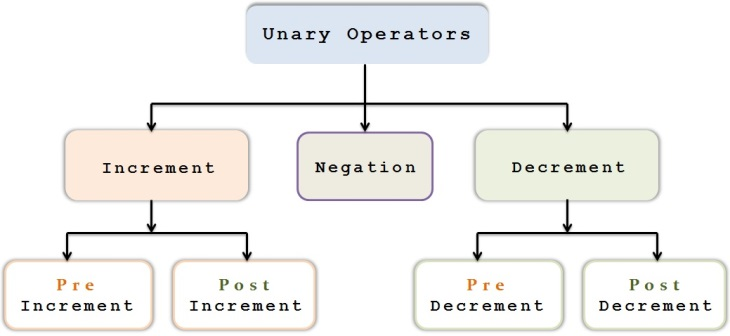###### Pre increment operator

This operator `increments `the value by one before its evaluation in the expression.

Example:

1. int x=5;
2. System.out.println(++x); // output will be 6
```int x=5;
System.out.println(++x); // output will be 6```

###### Pre decrement operator

This operator `decrements `the value by one before its evaluation in the expression.

Example:

1. int x=5;
2. System.out.println(--x); // output will be 4
```int x=5;
System.out.println(--x); // output will be 4```

###### Post increment operator

This operator `increments `the value by one after its evaluation in the expression.

Example:

1. int x=5;
2. System.out.println(x++); // output will be 5
3. System.out.println(x); // output will be 6 as its incremented after above line execution
```int x=5;
System.out.println(x++); // output will be 5
System.out.println(x); // output will be 6 as its incremented after above line execution```

###### Post decrement operator

This operator `decrements `the value by one after its evaluation in the expression.

Example:

1. int x=5;
2. System.out.println(x--); // output will be 5
3. System.out.println(x); // output will be 4 as its decremented after above line execution
```int x=5;
System.out.println(x--); // output will be 5
System.out.println(x); // output will be 4 as its decremented after above line execution```

###### Negation operator

It’s used to `invert` the Boolean value from true to false or false to true.

1. boolean success = false;
2.
3. System.out.println(success); output will be false
4.
5. System.out.println(!success); output will be true
```boolean success = false;

System.out.println(success); output will be false

System.out.println(!success); output will be true```

##### Arithmetic Operator

Arithmetic operators are used to perform basic arithmetic operations which are explained as below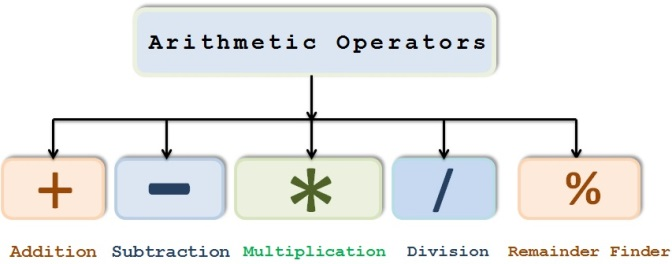It’s used to `add `the numbers or concatenation of strings

Example:

1. int num1=20;
2. int num2=10;
3. int result = num1+num2;
4. System.out.println(result); output will be 30
```int num1=20;
int num2=10;
int result = num1+num2;
System.out.println(result); output will be 30```

###### Subtraction

It’s used to `subtract `the numbers

Example:

1. int num1=20;
2. int num2=10;
3. int result = num1-num2;
4. System.out.println(result); output will be 10
```int num1=20;
int num2=10;
int result = num1-num2;
System.out.println(result); output will be 10```

###### Multiplication

It’s used to `multiply `the numbers

Example:

1. int num1=20;
2. int num2=10;
3. int result = num1*num2;
4. System.out.println(result); output will be 200
```int num1=20;
int num2=10;
int result = num1*num2;
System.out.println(result); output will be 200```

###### Division

It’s used to perform `division` among the numbers

Example:

1. int num1=20;
2. int num2=10;
3. int result = num1/num2;
4. System.out.println(result); output will be 2
```int num1=20;
int num2=10;
int result = num1/num2;
System.out.println(result); output will be 2```

###### Remainder Finder

It’s used to `divide` one number by another and returns the `remainder `as it’s result

Example:

1. int num1=20;
2. int num2=6;
3. int result = num1%num2;
4. System.out.println(result); output will be 2
```int num1=20;
int num2=6;
int result = num1%num2;
System.out.println(result); output will be 2```

We know that when we divide 20 by 6 , we get reminder as 2.

`Note The `+ `operator can also be used for concatenating (joining) two strings together`
1.         String firstString = "This is";
2.         String secondString = " a concatenated string.";
3.         String thirdString = firstString+secondString;
4.         System.out.println(thirdString); // output will be “This is a concatenated string”
```        String firstString = "This is";
String secondString = " a concatenated string.";
String thirdString = firstString+secondString;
System.out.println(thirdString); // output will be “This is a concatenated string”```

##### Relational Operator

Java has 6 relational operators that compare two operands and return a boolean value.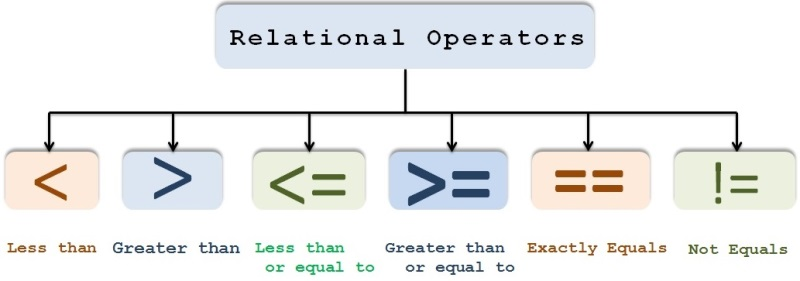##### Let us say `x `and `y `are the 2 operands

`x < y` is true if x is less than y, false otherwise

`x > y` is true if x is greater than y, false otherwise.

`x <= y` is true if x is less than or equal to y, false otherwise.

`x >= y` is true if x is greater than or equal to y, false otherwise.

`x == y` is true if x equals y, false otherwise.

`x != y` is true if x is not equal to y, false otherwise.

Example

1. boolean res1 = 10 < 20;  // true. As 10 is less than 20
2.
3. Boolean res2 = 10 > 20;  // false. As 10 is not greater than 20
4.
5. boolean res3 = 10 != 20;  // true. As 10 does not equal to 20
6.
7. boolean res4 = 10 >= 20; //false. As 10 is neither equal to 20 nor greater than 20
8.
9. boolean res5 = 10 <= 20; // true. As 10 is less than 20
10.
11. boolean res6 = 10 == 10; // true. As 10 is equal to 10
```boolean res1 = 10 < 20;  // true. As 10 is less than 20

Boolean res2 = 10 > 20;  // false. As 10 is not greater than 20

boolean res3 = 10 != 20;  // true. As 10 does not equal to 20

boolean res4 = 10 >= 20; //false. As 10 is neither equal to 20 nor greater than 20

boolean res5 = 10 <= 20; // true. As 10 is less than 20

boolean res6 = 10 == 10; // true. As 10 is equal to 10```

Generally, We use these conditions to check certain business condition and decide the flow based on that Boolean value.

Example

1. int age ;
2. If(age >= 18){
3. System.out.println(“Eligible for voting”);
4. }
5. Else{
6. System.out.println(“Not eligible for voting”);
7. }
```int age ;
If(age >= 18){
System.out.println(“Eligible for voting”);
}
Else{
System.out.println(“Not eligible for voting”);
}```

##### Logical operators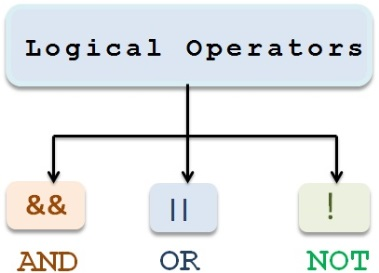###### Logical AND

This operator checks 2 operands

If both the operands are true then only it returns true.

If any one of them is false, then it returns false.

If first operand is false, then it won’t evaluate second operand,rather it returns whole result as false.

Example:

1. int a=20;
2. int b=10;
3. int c=30;
4. System.out.println(a<b&&a<c);//false && true  and hence result is false
```int a=20;
int b=10;
int c=30;
System.out.println(a<b&&a<c);//false && true  and hence result is false```

1. int a=5;
2. int b=10;
3. int c=30;
4. System.out.println(a<b&&a<c);//true && true  and hence result is true
```int a=5;
int b=10;
int c=30;
System.out.println(a<b&&a<c);//true && true  and hence result is true```

###### Logical OR

This operator checks 2 operands

If any one of the operands is true then it returns true.

If both of them are false, then it returns false.

If first operand is true, then it won’t evaluate second operand,rather it returns whole result as true.

Example:

1. int a=20;
2. int b=10;
3. int c=30;
4. System.out.println(a<b || a<c);//false || true  and hence result is true
```int a=20;
int b=10;
int c=30;
System.out.println(a<b || a<c);//false || true  and hence result is true```

1. int a=100;
2. int b=50;
3. int c=30;
4. System.out.println(a<b || b<c);//false || false  and hence result is false
```int a=100;
int b=50;
int c=30;
System.out.println(a<b || b<c);//false || false  and hence result is false```

###### Logical NOT

This operator is a unary logical operator which will just negate the boolean result.

Returns true if the operand to the right evaluates to false.

Returns false if the operand to the right is true.

Example:

1. boolean a=true;
2. !a returns false
```boolean a=true;
!a returns false```

1. boolean a=false;
2.   !a returns true
```boolean a=false;
!a returns true```

##### Practical use of Logical NOT is as below

1. Public Boolean isEligibleForVote(Person p){
2. If(p.age >= 18){
3.                  return true;
4.                }
5. }
6. If(!isEligibleForVote(p)){
7.       System.out.println(“You are not eligible for voting”);
8. }
```Public Boolean isEligibleForVote(Person p){
If(p.age >= 18){
return true;
}
}
If(!isEligibleForVote(p)){
System.out.println(“You are not eligible for voting”);
}```

##### Bitwise operators

Bitwise Shift operators are used to change individual bits in an operand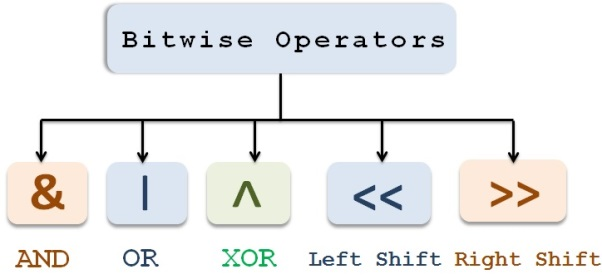##### Let’s see the below table to understand the results of bitwise &,| and ^

O can be treated as false

1 can be treated as true

`Bitwise " & "` returns true if both operands are true

`Bitwise " | "` returns true if any one of the operands is true

`Bitwise " ^ "` returns false if both the operands are true or both the operands are false.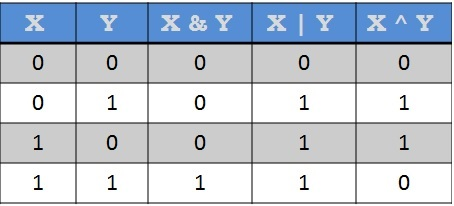##### Difference between Bitwise and Logical operators

###### Bitwise `& `and Logical `&&`

Bitwise `& `checks both the operands no matter whether first operand is true or false

Logical `&&` checks second operand only if first operand is true.

If first operand is `false` then it won’t evaluate second operand instead returns the result as `false`.

###### Bitwise `|` and Logical `||`

Bitwise `|` checks both the operands no matter whether first operand is true or false

Logical `|` checks second operand only if first operand is false.

If first operand is `true `then it won’t evaluate second operand instead returns the result as `true`

###### Bitwise shift operators

The bitwise shift operators shifts the `bit `value.

The left operand specifies the value to be shifted and the right operand specifies the number of positions that the bits in the value have to be shifted.

Example

1. a=0000100
2. b=3
3. a<<b left shifts the value in ‘a’ by 3 positions
4. a<<b = 0100000
5.
6. a>>b right shifts the value in ‘a’ by 3 positions
7. a>>b = 1000000
```a=0000100
b=3
a<<b left shifts the value in ‘a’ by 3 positions
a<<b = 0100000

a>>b right shifts the value in ‘a’ by 3 positions
a>>b = 1000000```

##### Assignment operators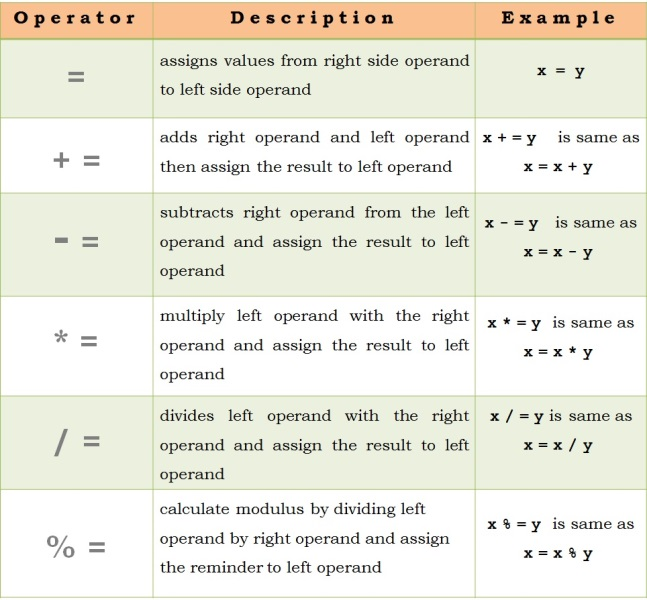Examples

1. int x=20;
2. int y=30;
3. x+=y;//x=x+y (x=20+30 and hence x=50)
4. System.out.println(x);
5. System.out.println(y);
```int x=20;
int y=30;
x+=y;//x=x+y (x=20+30 and hence x=50)
System.out.println(x);
System.out.println(y);  ```
1. int x=20;
2. int y=30;
3. x-=y;//x=x-y (x=20-30 and hence x=-10)
4. System.out.println(x);
5. System.out.println(y);
```int x=20;
int y=30;
x-=y;//x=x-y (x=20-30 and hence x=-10)
System.out.println(x);
System.out.println(y);  ```

##### Conditional operators

Conditional operator is represented by ? : which is a shorthand for if then else statement

This operator is also known as the ternary operator because it uses three operands.

Syntax :

1. result = someCondition ? value1 : value2;
`result = someCondition ? value1 : value2;`

If someCondition is true, assign the value of value1 to result. Otherwise, assign the value of value2 to result.

Example

1. int x=10;
2. int y=20;
3. int max=(x>y)?x:y;
4. System.out.println(“Max value is  “+max);
```int x=10;
int y=20;
int max=(x>y)?x:y;
System.out.println(“Max value is  “+max);```

Same condition can be written using if else as below

1. int x=10;
2. int y=20;
3. int max;
4. If(x>y){
5. Max=x;
6. }
7. Else{
8. Max=y;
9. }
10. System.out.println(“Max value is  “+max);
```int x=10;
int y=20;
int max;
If(x>y){
Max=x;
}
Else{
Max=y;
}
System.out.println(“Max value is  “+max);```

##### Instanceof operator

It’s a type comparison operator which compares an object to a specified type

We can use it to test if an object is an instance of a class, an instance of a subclass, or an instance of a class that implements a particular interface.

Example :

We have defined a parent class (named Parent), a simple interface (named MyInterface), and a child class (named Child) that inherits from the parent and implements the interface

1. interface MyInterface {
2.
3. }
```interface MyInterface {

}```

1. class Parent {
2.
3. }
```class Parent {

}```

1. class Child extends Parent implements MyInterface {
2.
3. }
```class Child extends Parent implements MyInterface {

}```

1. class InstanceofDemo {
2.     public static void main(String[] args) {
3.
4.        Parent obj1 = new Parent();
5.        Parent obj2 = new Child(); // Upcasting
6.
7.         System.out.println("obj1 instanceof Parent: "
8.             + (obj1 instanceof Parent));
9.         System.out.println("obj1 instanceof Child: "
10.             + (obj1 instanceof Child));
11.         System.out.println("obj1 instanceof MyInterface: "
12.             + (obj1 instanceof MyInterface));
13.         System.out.println("obj2 instanceof Parent: "
14.             + (obj2 instanceof Parent));
15.         System.out.println("obj2 instanceof Child: "
16.             + (obj2 instanceof Child));
17.         System.out.println("obj2 instanceof MyInterface: "
18.             + (obj2 instanceof MyInterface));
19. }
20. }
```class InstanceofDemo {
public static void main(String[] args) {

Parent obj1 = new Parent();
Parent obj2 = new Child(); // Upcasting

System.out.println("obj1 instanceof Parent: "
+ (obj1 instanceof Parent));
System.out.println("obj1 instanceof Child: "
+ (obj1 instanceof Child));
System.out.println("obj1 instanceof MyInterface: "
+ (obj1 instanceof MyInterface));
System.out.println("obj2 instanceof Parent: "
+ (obj2 instanceof Parent));
System.out.println("obj2 instanceof Child: "
+ (obj2 instanceof Child));
System.out.println("obj2 instanceof MyInterface: "
+ (obj2 instanceof MyInterface));
}
}```

`NoteWhen using the instanceof operator with null , it returns false as null is not an instance of anything.`